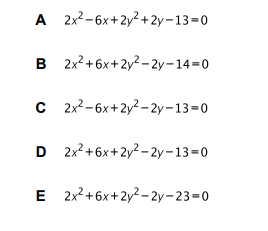# IMAT 2013 Q56 [Equation of a Circle]

Which of the following is the equation of the circle with centre (­-1.5, 0.5) and radius 3?The equation of the circle is (x-x_1)^2 + (y-y_1)^2 = r^2. In which the centre of the circle is given as (x_1,y_1) and r is the radius of the circle. Having that in mind, we can continue to solve the problem:
In the text it is said that the centre of the circle is (­-1.5, 0.5) and radius is 3, meaning x_1=-1.5 , y_1 = 0.5 , r=3. Putting that information in the formula:

(x-x_1)^2 + (y-y_1)^2 = r^2 \to (x-(-1.5))^2 + (y-0.5)^2 = 3^2 \to (x+\frac{3}{2})^2 + (y-\frac{1}{2}))^2 = 9

Using the binomial theorem, we get x^2 + 2\cdot \frac{3}{2}x + (\frac{3}{2})^2 + y^2 - 2\cdot\frac{1}{2}y + \frac{1}{2}^2 =9 \to x^2 + 3x + \frac{9}{4} + y^2 - y + \frac{1}{4}=9
\to x^2 + 3x + y^2 - y + \frac{10}{4} - 9 = 0 \to x^2 + 3x + y^2 - y + \frac{5}{2} - 9 = 0
Multiplying the whole equation by 2 gives us: 2x^2 + 6x + 2y^2 -2 y + 5 - 18 = 0 \to 2x^2 + 6x + 2y^2 -2 y -13= 0.